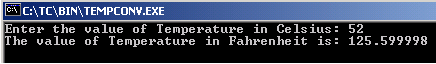Tutorials

# C Temperature Converter

Here we are going to illustrates you how to convert temperature from Celsius to Fahrenheit in C.

Here we are going to illustrates you how to convert temperature from Celsius to Fahrenheit in C.

# C Temperature Converter

Here we are going to illustrates you how to convert temperature from Celsius to Fahrenheit in C. You can see in the given example, we prompt the user to enter the temperature in celsius. The scanf() function gets the entered temperature and by using the following statement shown, the temperature is converted into Fahrenheit

fahrenheit = (1.8 * celcius) + 32;

Here is the code:

TEMPCONV.C

 ``` #include  #include  int main() {   float celcius, fahrenheit;   printf("Enter the value of Temperature in Celsius: ");   scanf("%f", &celcius);   fahrenheit = (1.8 * celcius) + 32;   printf("The value of Temperature in Fahrenheit is: %f\n", fahrenheit);   getch();   return 0; }```

Output will be displayed as:

TEMPCONV.EXE